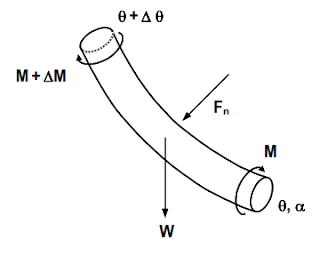# DRILL STRING TORQUE DEFINITION & CALCULATIONS

### Drill Pipe Torque Calculations

We can define the drill string torque,as it is the rotational force that is required to overcome all the frictional forces between the drill string and the formation while drill string rotation.

The torque required to turn the drill string is calculated by the following equation,

Where
T       =       Torque, ft-lb
Fn     =       normal force as defined
R        =       Radius of drill string component ft (for drill collars use outer radius of collar,
for DP, HWDP and casing use outer radius of tool joint)
µ       =       Coefficient of friction
A      =       Angular speed
V      =       Resultant speedFrom drill string torque definition, If the drill string is not tripped while rotating, then T = 0 and A is equal to V and The Equation reduces to
Torque = Fn

If the torque acting on the lower end of the drill string element is M, then the torque acting at the top of the element is M + ΔM . The torque increment ΔM is

ΔM = FnRµ

where Fn  is the normal force exerted on the element.

The torque required to turn the entire drill string is the sum of all torque increments for all the elements.

### Example On The Drill String Torque Calculations

Given:

#### Well bore

9-5/8” 40# casing at 5000 ft, Total Depth = 10,000 ft, Hole Size = 8-1/2”, Kick off Point = 5000 ft
Build Rate = 10 deg/100 ft, End of Build at = 5600 ft, Tangent Section = 5600 to 10,000
Inclination across tangent section = 60 deg, Azimuth = 0 deg north (surface to 5000 ft)
Azimuth is changed at the rate of 5 deg / 100 ft from 5000 ft to 5600 ft
Azimuth = 30 deg from 5600 ft to 10,000 ft
Mud Weight = 90 pcf, Coefficient of friction = 0.25 in open hole and 0.2 in casing.

#### Drill String

Drill pipe 5” OD, 5.276”ID, 19.5# normal weight 20.89# adjusted weight
Drill Collars 7” OD, 2.25” ID, 117.42#, 400 ft long

With the drill string at bottom, calculate:

a) Calculate the surface torque required to turn the string off bottom with no tripping.
b) Calculate the torque while drilling. Use a bit torque of 2000 ft-lb. Drill pipe tool  joint OD is 6.625 in.

Solution

The drill string is divided into small elements as was done for calculating drag.
Calculations are tabulated in below Table. The data in columns 10, 11 and 13 are calculated in the same manner as was done for the drag calculations in the previous example.

For drag calculations the tension increment Δ F in column 12 is calculated by below Eq.

where µFn in the incremental drag caused by moving the element up hole. However, since in this example there is no tripping, the drag increment due to uphole or downhole movement is zero, and the tension increment Δ Ft is

Column 14 is the torque at the bottom of the element. If the string is off bottom the torque is zero. For the case of drilling the torque is the bit torque which have to be estimated. The torque increment Δ M  in Column 15 is the torque resistance exerted by the element and is calculated by using below Eq.
ΔM = FnRµ

Column 16 is the torque at the top of the element which is M + Δ M. The torque at the top of the element ( M + Δ M ) is the torque at the bottom of the next element. The calculations are repeated for all elements to the surface.

Calculations for Element # 1

Columns 2 through 11 are calculated as in the previous example.

Column # 12. is calculated
Δ Ft = 38278 x cos60 = 19139 lb

Column # 14. The torque at bottom of element in this case is zero because the drill string is off bottom (no bit torque)

Column # 15. Torque increment Δ M
Δ M = Fnµr

Drill Collar OD
r = ------------------------ = 7/2 = 3.5" = 0.2916 ft
2

Δ M = 33149 x 0.25 x 0.2916 = 2416 ft-lb

Column # 16. Torque at top of element #1 is M + Δ M = 0 + 2416 ft-lb = 2416 ft-lb

Calculation for element # 2

Columns # 10 and 11 are calculated in same manner as in the drag example

Column # 12:
ΔF = 68101 cos 60 = 34050 lb

Column # 14: Torque at bottom of element # 2 is same as torque at top of element # 1 or 2416 ft-lb

Column # 15:
Δ M = Fnrµ

Tool Joint OD
r = ------------------------ = 6.625/2 = 3.312" = 0.276 ft
2

Δ M = 58977 x 0.276 x 0.25 = 4069 ft - lb

Column # 16: Torque at top of element # 2 is

Δ M + M = 4069 + 2416 = 6485 ft-lb

Calculations for elements 3 through 14 are done in the same manner as for element # 2.

For element # 15
Since the inclination angle is zero, the normal force F is zero and the torque increment,
Δ M is also zero. In other words, drill pipe in vertical hole can be turned with no or very little torque. So the torque at top of element # 15 (the surface) is equal to torque at top of element #14 which is 11032 ft-lb

b) If the bit torque is 2000 ft-lb then the torque at surface is

Torque = 11032 + 2000 = 13032 ft-lb

It can be seen from the above example problems that the calculation of torque and drag consumes large amount of time and is best done by using computer software.